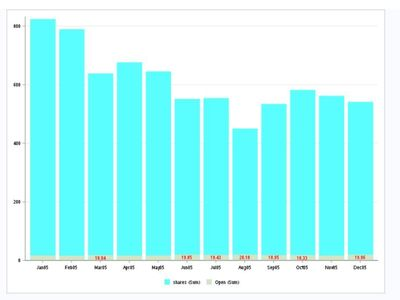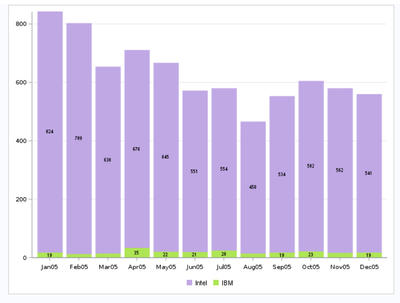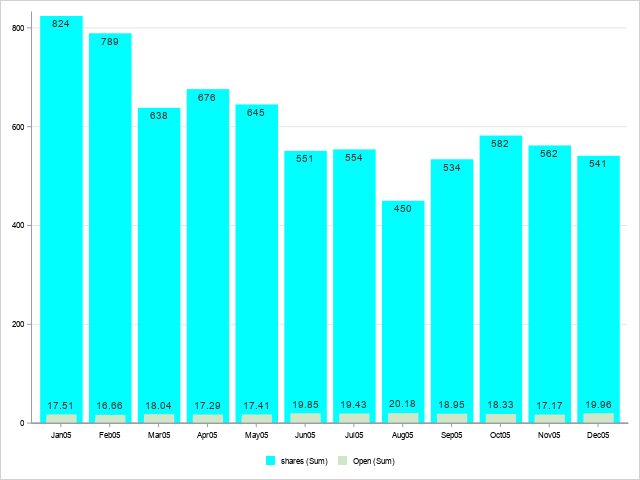## Stacked vbar seglabel value not displayed when value too small

I would like to display all datalabels of the smaller segment of a vbar chart.  However, when the values are too small, the labels are getting supressed.  My questions are:

1. Is there a way to force the values to be displayed not matter how small?

2. Is there a way I can place the label above the smaller bars?

I am using SAS EG 7.1 where the SAS engine is 9.4 (rel 3)

Please note I am using sample data just to illustrate my request.

Thanks!

``````data test;
set sashelp.stocks;
where year(date)=2005 and stock="Intel";
shares=int(volume/100000);
open=open/1.35;
run;

proc sgplot noborder data=test;

styleattrs  datacolors=( CYAN PALG);
vbar date/ response=shares nooutline;
vbar date/ response=open nooutline seglabel seglabelattrs=(size=6pt color=red)   ;
xaxis fitpolicy=thin display=(nolabel) valueattrs=(size=6 color=black);
yaxis grid display=(nolabel) valueattrs=(size=6 color=black);
keylegend / location=outside position=bottom noborder valueattrs=(size=6) autooutline;
format date monyy. open 5.2;
run;
``````1 ACCEPTED SOLUTION

Accepted Solutions

## Re: Stacked vbar seglabel value not displayed when value too small

Or, to put the label on top of the short bar ...

data anno_text; set test;
length label \$100 anchor x1space y1space function \$50 textcolor \$12;
function='text';
x1=date; y1=open;
x1space='datavalue'; y1space='datavalue';
anchor='bottom';
textcolor="cxff0000"; textsize=6; textweight='normal';
width=100; widthunit='percent';
label=trim(left(put(open,comma8.2)));
run;

Also note, you're not really using 'stacked' bars as your title implies (where the height of the 2 bars adds together). I think you're actually overlaying the short bars in front of the taller bars. If you're wanting stacked bars, then you'll need to do something like change your data a bit, and use a group= and groupdisplay=stack.

8 REPLIES 8

## Re: Stacked vbar seglabel value not displayed when value too small

I think the best solution is to make 2 charts. But if you want to keep both in one, you can use y2axis, to make the 2 charts use different y-axis.

``````  proc sgplot noborder data=test;

styleattrs  datacolors=( CYAN PALG);
vbar date/ response=shares nooutline;
vbar date/ y2axis response=open nooutline seglabel seglabelattrs=(size=6pt color=red)   ;
xaxis fitpolicy=thin display=(nolabel) valueattrs=(size=6 color=black);
yaxis grid display=(nolabel) valueattrs=(size=6 color=black);
keylegend / location=outside position=bottom noborder valueattrs=(size=6) autooutline;
format date monyy. open 5.2;
run;``````

Of course you have to make them transparent or increase the max of your y2axis to display both charts.

## Re: Stacked vbar seglabel value not displayed when value too small

However, my client requires the scale be kept the same for both bars.  It's not necessary for small var to be visible as long as the data label is displayed.

Thanks!

## Re: Stacked vbar seglabel value not displayed when value too small

I don't think you can annotate on specific stacked bar segments, but if you know this small bar segment is always going to be at the bottom of the bar, you could annotate the text label at the bottom of the bar, something like this...

data test; set sashelp.stocks;
where year(date)=2005 and stock="Intel";
shares=int(volume/100000);
open=open/1.35;
run;

data anno_text; set test;
length label \$100 anchor x1space y1space function \$50 textcolor \$12;
function='text';
x1=date; y1=0;
x1space='datavalue'; y1space='datapercent';
anchor='bottom';
textcolor="cxff0000"; textsize=6; textweight='normal';
width=100; widthunit='percent';
label=trim(left(put(open,comma8.2)));
run;

proc sgplot noborder data=test sganno=anno_text;
styleattrs datacolors=( CYAN PALG);
vbar date/ response=shares nooutline;
vbar date/ response=open nooutline;
xaxis fitpolicy=thin display=(nolabel) valueattrs=(size=6 color=black);
yaxis grid display=(nolabel) valueattrs=(size=6 color=black);
keylegend / location=outside position=bottom noborder valueattrs=(size=6) autooutline;
format date monyy. open 5.2;
run;## Re: Stacked vbar seglabel value not displayed when value too small

Hi Robert,

I hope it's OK to ask a follow-up question.  Suppose I also have a true stacked vbar, as in the example below.  You mentioned previously I need to modify the data bit in such cases.  Could you please clarify whether I should split up the data (stock) into two separate variables (IBM and Intel) and then treat the problem exactly as in my previous question?  Or is there another method?

Following is my current code and output, where I would like to display the values for all of the green segments.

``````data test;
set sashelp.stocks (where= (year(date)=2005));
if stock IN ("Intel","IBM");
shares=int(volume/100000);
if stock="IBM" then shares=int(shares/3);
run;

proc sgplot noborder data=test;
vbar date /response=shares seglabelattrs=(size=6pt)
group=stock  seglabel GROUPORDER=ascending nooutline;
styleattrs  datacolors=(BIGY VPAPB) ;
xaxis fitpolicy=thin display=(nolabel)
valueattrs=(size=7 color=black Family=Arial);
yaxis grid display=(nolabel)
valueattrs=(size=7 color=black Family=Arial) valueshint min=0 offsetmax=0 fitpolicy=thin;
keylegend "VBAR" / title="" location=outside position=bottom noborder
valueattrs=(size=8) SORTORDER= descending  noopaque autooutline;

format  date monyy. shares 4.0;
run;``````

Thanks!## Re: Stacked vbar seglabel value not displayed when value too small

Or, to put the label on top of the short bar ...

data anno_text; set test;
length label \$100 anchor x1space y1space function \$50 textcolor \$12;
function='text';
x1=date; y1=open;
x1space='datavalue'; y1space='datavalue';
anchor='bottom';
textcolor="cxff0000"; textsize=6; textweight='normal';
width=100; widthunit='percent';
label=trim(left(put(open,comma8.2)));
run;

Also note, you're not really using 'stacked' bars as your title implies (where the height of the 2 bars adds together). I think you're actually overlaying the short bars in front of the taller bars. If you're wanting stacked bars, then you'll need to do something like change your data a bit, and use a group= and groupdisplay=stack.

## Re: Stacked vbar seglabel value not displayed when value too small

Thanks Robert for both the options. You are right, it's not a stacked bar in the true sense, more of an overlay. I am in the process of applying each of your technique and will present both to my client. My preferred one is the second option but it's not my choice which one will get picked.

## Re: Stacked vbar seglabel value not displayed when value too small

Since these aren't really 'stacked' bars, I would recommend making the shorter bar more narrow than the taller one (so it's more intuitive that they are overlaid, not stacked). I've also added code to annotate a label above the top of the short bar, and below the top of the tall bar...

data test; set sashelp.stocks;
where year(date)=2005 and stock="Intel";
shares=int(volume/100000);
open=open/1.35;
run;

data anno_text; set test;
length label \$100 anchor x1space y1space function \$50 textcolor \$12;
function='text'; layer='front';
x1=date; x1space='datavalue'; y1space='datavalue';
textcolor="gray33"; textsize=7; textweight='normal';
width=100; widthunit='percent';
y1=open; anchor='bottom';
label=trim(left(put(open,comma8.2)));
output;
y1=shares; anchor='top';
label=trim(left(put(shares,comma8.0)));
output;
run;

proc sgplot noborder data=test sganno=anno_text;
styleattrs datacolors=( CYAN PALG);
vbar date/ response=shares nooutline;
vbar date/ response=open nooutline barwidth=.6;
xaxis fitpolicy=thin display=(nolabel) valueattrs=(size=6 color=black);
yaxis grid display=(nolabel) valueattrs=(size=6 color=black);
keylegend / location=outside position=bottom noborder valueattrs=(size=6) autooutline;
format date monyy. open 5.2;
run;## Re: Stacked vbar seglabel value not displayed when value too small

Thanks Robert, that is a nice workaround for an overlay bar chart.

However, if it is a real stacked bar chart as in the example in my followup question in this link, could you suggest a solution please

https://communities.sas.com/t5/Graphics-Programming/Stacked-vbar-seglabel-value-not-displayed-when-v...

Thanks.

Discussion stats
• 8 replies
• 1861 views
• 4 likes
• 3 in conversation